A practical algorithm for determining if there exist integers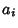for given real numberssuch thator else establish bounds within which no such Integer Relation can exist (Ferguson and Forcade 1979). A nonrecursive variant of the original algorithm was subsequently devised by Ferguson (1987). The Ferguson-Forcade algorithm has shown that there are no algebraic equations of degreewith integer coefficients having Euclidean norms below certain bounds for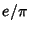,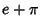,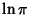,,,,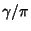, and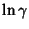, where e is the base for the Natural Logarithm,is Pi, andis the Euler-Mascheroni Constant (Bailey 1988).

 Constant Bound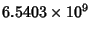References

Bailey, D. H. Numerical Results on the Transcendence of Constants Involving,, and Euler's Constant.'' Math. Comput. 50, 275-281, 1988.

Ferguson, H. R. P. A Short Proof of the Existence of Vector Euclidean Algorithms.'' Proc. Amer. Math. Soc. 97, 8-10, 1986.

Ferguson, H. R. P. A Non-Inductive GL() Algorithm that Constructs Linear Relations for-Linearly Dependent Real Numbers.'' J. Algorithms 8, 131-145, 1987.

Ferguson, H. R. P. and Forcade, R. W. Generalization of the Euclidean Algorithm for Real Numbers to All Dimensions Higher than Two.'' Bull. Amer. Math. Soc. 1, 912-914, 1979.